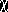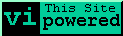# Real Time Distribution of Scores for the Continuing Turing Test of the Fractal RealizerThe population of Turing test scores is shown as an histogram above. A2 goodness-of-fit test of the population of scores against a binomial where p = q = 0.50 is shown as the green, leftmost curve in the histogram. The random binomial distribution (yellow, middle curve) is symmetrical about the 0.5 mean. A2 goodness-of-fit test of the population of scores against a binomial where p = 0.6 and q = 0.4 is shown as the red, rightmost curve in the histogram.GEOBABBLE!